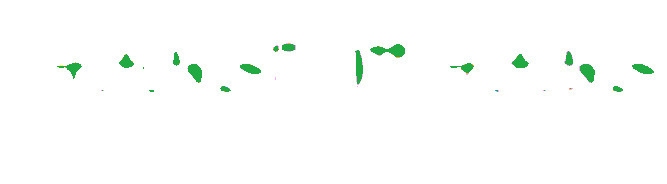# Wand mode() function – Python

• Last Updated : 19 May, 2021

The mode() function is an inbuilt function in the Python Wand ImageMagick library which is used to replace each pixel with the mathematical mode of the neighboring colors.

Syntax:

Attention geek! Strengthen your foundations with the Python Programming Foundation Course and learn the basics.

To begin with, your interview preparations Enhance your Data Structures concepts with the Python DS Course. And to begin with your Machine Learning Journey, join the Machine Learning - Basic Level Course

`mode(width, height)`

Parameters: This function accepts two parameters as mentioned above and defined below:

• width: This parameter is used to store the number of neighboring pixels to include in mode.
• height: This is an optional parameter is used to store height of neighboring pixels. By default it is same as width.

Return Value: This function returns the Wand ImageMagick object.

Original Image:Example 1:

## Python3

 `# Import library from Image``from` `wand.image ``import` `Image` `# Import the image``with Image(filename ``=``'../geeksforgeeks.png'``) as image:``    ``# Clone the image in order to process``    ``with image.clone() as mode:``        ``# Invoke mode function with width as 30 and height as 10``        ``mode.mode(``30``, ``10``)``        ``# Save the image``        ``mode.save(filename ``=``'mode1.jpg'``)`

Output:Example 2:

## Python3

 `# Import libraries from the wand ``from` `wand.image ``import` `Image``from` `wand.drawing ``import` `Drawing``from` `wand.color ``import` `Color` `with Drawing() as draw:``    ``# Set Stroke color the circle to black``    ``draw.stroke_color ``=` `Color(``'black'``)``    ``# Set Width of the circle to 2``    ``draw.stroke_width ``=` `1``    ``# Set the fill color to 'White (# FFFFFF)'``    ``draw.fill_color ``=` `Color(``'white'``)` `    ``# Invoke Circle function with center at 50, 50 and radius 25``    ``draw.circle((``200``, ``200``), ``# Center point``                ``(``100``, ``100``)) ``# Perimeter point``    ``# Set the font style``    ``draw.font ``=` `'../Helvetica.ttf'``    ``# Set the font size``    ``draw.font_size ``=` `30``    ` `    ``with Image(width ``=` `400``, height ``=` `400``, background ``=` `Color(``'# 45ff33'``)) as pic:``        ``# Set the text and its location``        ``draw.text(``int``(pic.width ``/` `3``), ``int``(pic.height ``/` `2``), ``'GeeksForGeeks !'``)``        ``# Draw the picture``        ``draw(pic)``        ``# Invoke mode function with width and height as 200``        ``pic.mode(``200``)``        ``# Save the image``        ``pic.save(filename ``=``'mode2.jpg'``)`

Output:My Personal Notes arrow_drop_up